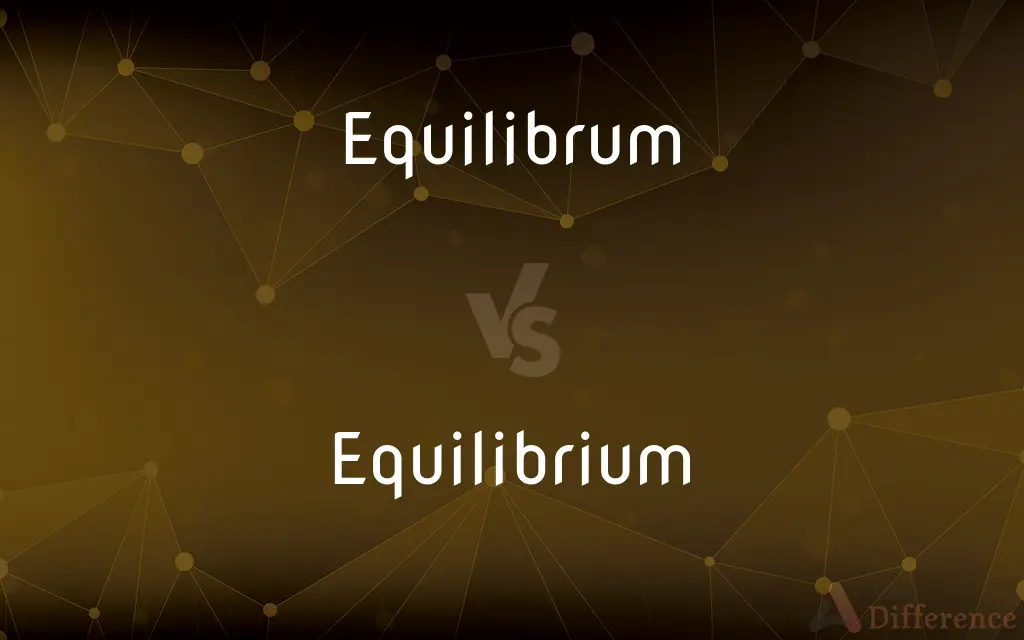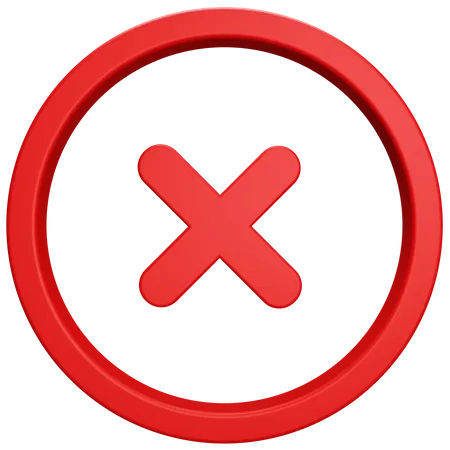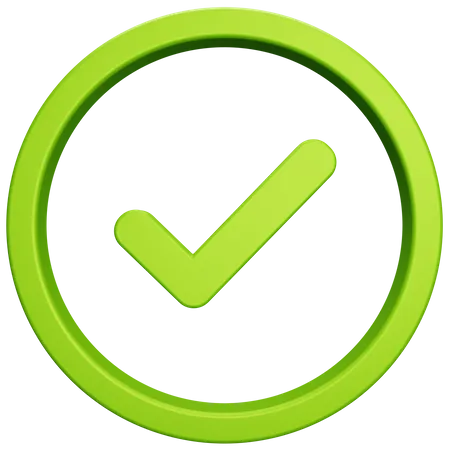# Equilibrum vs. Equilibrium — What's the Difference?

"Equilibrum" is the incorrect spelling. The correct spelling is "Equilibrium," which refers to a state of balance or stability.## Which is correct: Equilibrum or Equilibrium

How to spell Equilibrium?### Equilibrum

Incorrect SpellingCorrect Spelling

## Key Differences

"Equilibrium" has the extra "i" before the "u."
Think of "equal" balance, but with an extra "i" for "Equilibrium."
Recall: “It takes equal effort to find the right equilibrium.”
Visualize: "Balance the 'I' in Equilibrium."
Use mnemonic: "EQUal In LIBerty RIghtly Means Equilibrium."

## Compare with Definitions

#### Equilibrum

Equilibrum is an incorrect spelling of Equilibrium.

#### Equilibrium

In chemistry, a state in which reactions occur at the same rate.
The reaction reached chemical equilibrium.

#### Equilibrium

A calm mental state, emotional balance.
She maintained her equilibrium under pressure.

#### Equilibrium

In physics, a condition in which a system is stable.
The pendulum is in equilibrium.

#### Equilibrium

Balance between supply and demand.
The equilibrium price was determined.

#### Equilibrium

A condition in which all acting influences are canceled by others, resulting in a stable, balanced, or unchanging system.

#### Equilibrium

Mental or emotional balance.

#### Equilibrium

(Physics) The state of a body or physical system at rest or in unaccelerated motion in which the resultant of all forces acting on it is zero and the sum of all torques about any axis is zero.

#### Equilibrium

The state of a chemical reaction in which its forward and reverse reactions occur at equal rates so that the concentration of the reactants and products does not change with time.

#### Equilibrium

The state of a system in which more than one phase exists and exchange between phases occurs at equal rates so that there is no net change in the composition of the system.

#### Equilibrium

The condition of a system in which competing influences are balanced, resulting in no net change.

Mental balance.

#### Equilibrium

(chemistry) The state of a reaction in which the rates of the forward and reverse reactions are the same.

#### Equilibrium

(physics) The state of a body at rest or in uniform motion in which the resultant of all forces on it is zero.

#### Equilibrium

Equality of weight or force; an equipoise or a state of rest produced by the mutual counteraction of two or more forces.

#### Equilibrium

A balancing of the mind between motives or reasons, with consequent indecision and doubt.

#### Equilibrium

Equality of distribution

#### Equilibrium

A sensory system located in structures of the inner ear that registers the orientation of the head

#### Equilibrium

A state in which opposing forces or influences are balanced.
The market reached equilibrium.

## Common Curiosities

#### Which vowel is used before Equilibrium?

"An" as in "an equilibrium."

#### Why is it called Equilibrium?

It's derived from Latin, meaning "even balance" (aequus meaning "equal" and libra meaning "balance").

#### What is the pronunciation of Equilibrium?

It's pronounced [ˌiːkwɪˈlɪbriəm].

#### Which preposition is used with Equilibrium?

"In" as in "in equilibrium."

Equilibria.

#### Which conjunction is used with Equilibrium?

No specific conjunction is exclusive to "Equilibrium."

#### What is the root word of Equilibrium?

The root words are Latin "aequus" (equal) and "libra" (balance).

#### What is the verb form of Equilibrium?

The verb form related to equilibrium is "equilibrate."

Yes.

Equilibrium.

It is a noun.

Neutral.

E-qui-lib-ri-um.

Noun.

#### Which article is used with Equilibrium?

"An" or "the" depending on the context.

#### Is the Equilibrium term a metaphor?

Not inherently, but can be used metaphorically in certain contexts.

#### What is the stressed syllable in Equilibrium?

The third syllable, "lib."

#### What is the opposite of Equilibrium?

Imbalance or disequilibrium.

#### What is the second form of Equilibrium?

Not applicable for nouns.

#### Which determiner is used with Equilibrium?

"This" as in "this equilibrium."

#### What is the first form of Equilibrium?

Equilibrium (as it is primarily a noun).

#### How is Equilibrium used in a sentence?

The market eventually finds its equilibrium between supply and demand.

No.

#### Is Equilibrium a vowel or consonant?

"Equilibrium" is a word, not a singular letter.

No.

#### Is Equilibrium a countable noun?

It can be, e.g., "different equilibria."

No.

Five syllables.

Balance.

#### What is the third form of Equilibrium?

Not applicable for nouns.